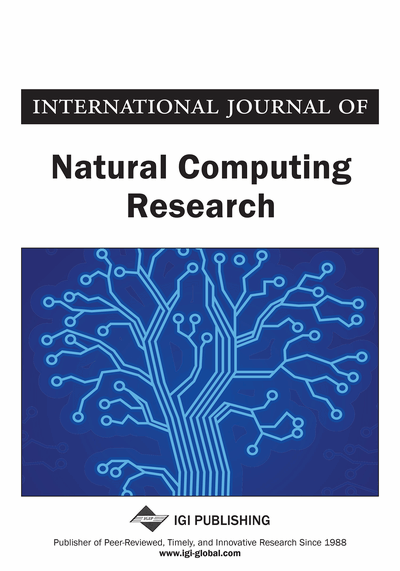# VRoptBees: A Bee-Inspired Framework for Solving Vehicle Routing Problems

Thiago A.S. Masutti (Mackenzie Presbyterian University, Sao Paulo, Brazil) and Leandro Nunes de Castro (Mackenzie Presbyterian University, Sao Paulo, Brazil)
DOI: 10.4018/IJNCR.2018010103
Available
\$37.50
No Current Special Offers

## Abstract

Combinatorial optimization problems are broadly studied in the literature. On the one hand, their challenging characteristics, such as the constraints and number of potential solutions, inspires their use to test new solution techniques. On the other hand, the practical application of these problems provides support of daily tasks of people and companies. Vehicle routing problems constitute a well-known class of combinatorial optimization problems, from which the Traveling Salesman Problem (TSP) is one of the most elementary ones. TSP corresponds to finding the shortest route that visits all cities within a path returning to the start city. Despite its simplicity, the difficulty in finding its exact solution and its direct application in practical problems in multiple areas make it one of the most studied problems in the literature. Algorithms inspired by biological phenomena are being successfully applied to solve optimization tasks, mainly combinatorial optimization problems. Those inspired by the collective behavior of insects produce good results for solving such problems. This article proposes the VRoptBees, a framework inspired by honeybee behavior to tackle vehicle routing problems. The framework provides a flexible and modular tool to easily build solutions to vehicle routing problems. Together with the framework, two examples of implementation are described, one to solve the TSP and the other to solve the Capacitated Vehicle Routing Problem (CVRP). Tests were conducted with benchmark instances from the literature, showing competitive results.
Article Preview
Top

## 1. Introduction

Optimization consists of determining the values of a set of variables that minimize or maximize a given mathematical expression, satisfying all problem constraints (Cunha et al., 2012; Michalewicz & Fogel, 2013). An intuitive way to solve a given optimization problem is to list all possible solutions, evaluate them, and use the best solution found. However, depending on the characteristics of the problem this approach, known as exhaustive search, is not efficient. The main drawback of using full enumeration is that it becomes computationally impractical depending on the number of possible solutions to the problem. This means that this exact solution approach would be valid only for simpler problems, what hardly occurs in practical applications.

The Vehicle Routing Problem (VRP) corresponds to a class of combinatorial optimization problems that seek for an optimal set of routes for a fleet of vehicles to traverse to attend a given set of customers (Lawler, 1985; Laporte, 1992; Reinelt, 1991; da Cunha, 2000; Toth & Vigo, 2001; Gutin & Punen, 2002; Laporte, 2009). As in most real cases the vehicles have a limited capacity, VRP becomes CVRP, which stands for Capacitated Vehicle Routing Problem. In mathematical terms, the CVRP can be described as follows. Given a number K of identical vehicles, each with a capacity Q, a depot id, a set S = {i1, i2, i3, ..., in} with n customers, each with a demand qi (i = 1, 2, 3, …, n), and the cost Cij (i,j = 1, 2, 3, ..., n + 1) of going from customer i to customer j and from each customer to the depot, the CVRP consists of determining the permutation π of the elements in S so as to minimize:

(1) subject to:(2) where πk,i represents the city visited in order i in route k, and nk is the number of customers visited by vehicle k. For a review of practical applications and solution algorithms the work of Laporte et al. (2000) and Laporte (2009) are recommended.

If the vehicle capacity and the demand of each customer (city) constraints are removed, then we have the Multiple Travelling Salesman Problem (MTSP), which is a particular case of the CVRP. Given a number M of salesman, a depot id, a set S = {i1, i2, i3, ..., in} with n intermediate cities, the cost Cij (i,j = 1, 2, 3, ..., n + 1) of going from one city to another and also from each city to the depot, the MTSP consists of determining the set of permutations πm (m = 1, 2, 3, …, M) from the elements in S so as to minimize:

(3) where πm,i represents the city visited in order i in route m, and nm is the number of intermediary cities visited in route m. The MTSP already presents enough characteristics to represent practical problems, such as school vehicle routing (Angel et al., 1972). Bektas (2006) presented other examples of practical applications and algorithms for MTSP.

## Complete Article List

Search this Journal:
Reset
Open Access Articles: Forthcoming
Volume 11: 4 Issues (2022): Forthcoming, Available for Pre-Order
Volume 10: 4 Issues (2021): 3 Released, 1 Forthcoming
Volume 9: 4 Issues (2020)
Volume 8: 4 Issues (2019)
Volume 7: 4 Issues (2018)
Volume 6: 2 Issues (2017)
Volume 5: 4 Issues (2015)
Volume 4: 4 Issues (2014)
Volume 3: 4 Issues (2012)
Volume 2: 4 Issues (2011)
Volume 1: 4 Issues (2010)
View Complete Journal Contents Listing